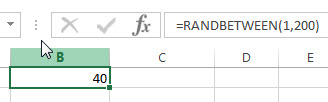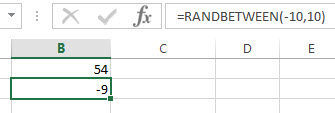# Excel RANDBETWEEN Function

This post will guide you how to use Excel RANDBETWEEN function with syntax and examples in Microsoft excel.

### Description

The Excel RANDBETWEEN function returns a random integer number that is between the numbers you specify. Each time when the worksheet is calculated or opened, a new random integer number is generated. So you can use the RANDBETWEEN function to generate a random integer number between tow given values.

The RANDBETWEEN function is a build-in function in Microsoft Excel and it is categorized as a Math and Trigonometry Function.

The RANDBETWEEN function is available in Excel 2016, Excel 2013, Excel 2010, Excel 2007, Excel 2003, Excel XP, Excel 2000, Excel 2011 for Mac.

### Syntax

The syntax of the RANDBETWEEN function is as below:

`= RANDBETWEEN (bottom,top)`

Where the RANDBETWEEN function arguments are:

• bottom -This is a required argument. The smallest integer number that RANDBETWEEN Function will return.
• top – This is a required argument. The largest integer number that RANDBETWEEN Function will return.

Note:

• If the bottom argument is greater than the given top value, the RANDBETWEEN function will return #NUM! Error.
• If either of the given arguments are non-numeric, this function will return #VALUE! Error.

### Excel RANDBETWEEN Function Examples

The below examples will show you how to use Excel RANDBETWEEN Function to generate a random integer between two supplied integer numbers.

1# get a random number between 1 and 200, enter the following formula in Cell B1.

`=RANDBETWEEN(1,200)`2# get a random number between -10 and 10, enter the following formula in Cell B2.

`=RANDBETWEEN(-10,10)`### Related Functions

• Excel RAND function
The Excel RAND function returns a random real number that is greater than or equal to 0 and less than 1. The syntax of the RAND function is as below:=RAND ()…

### More Excel Randbetween Function Examples

• Generate Random Character Strings
To generate random character strings in excel, you should be generate random numbers, letters, and special characters. We have talked that how to generate a random number string with Excel RANDBETWEEN function in excel…